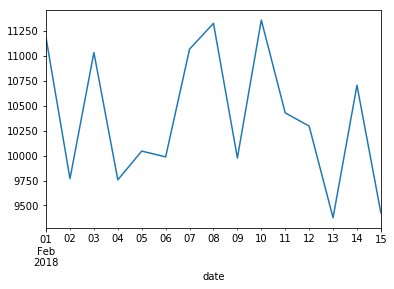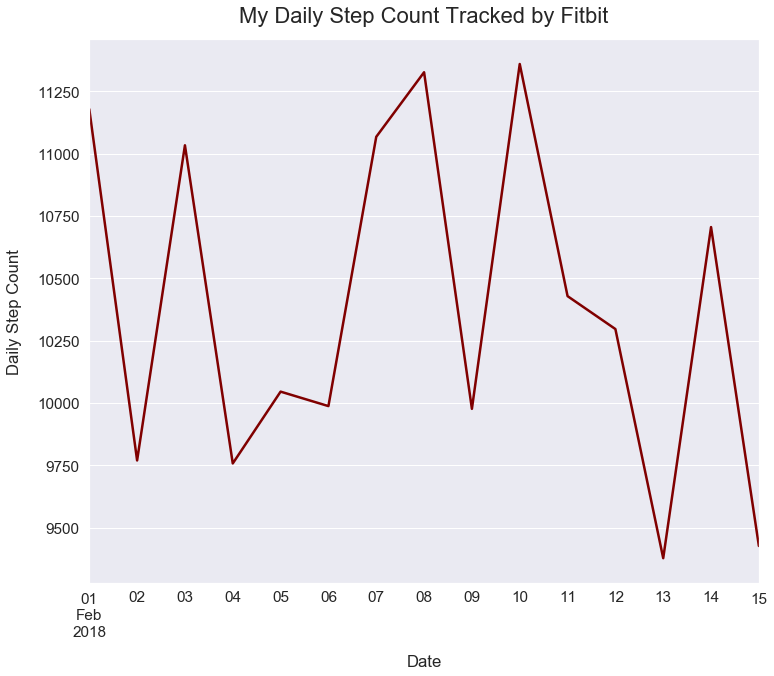Data Visualizations Pandas Plot Tutorial

# Line Plot using Pandas

#### Import Modules¶

In :
import matplotlib.pyplot as plt
import pandas as pd
import seaborn as sns
% matplotlib inline


#### Generate a Line Plot from My Fitbit Activity Data¶

More often, you'll be asked to generate a line plot to show a trend over time.

Below is my Fitbit activity of steps for each day over a 15 day time period.

In :
dates = ['2018-02-01', '2018-02-02', '2018-02-03', '2018-02-04',
'2018-02-05', '2018-02-06', '2018-02-07', '2018-02-08',
'2018-02-09', '2018-02-10', '2018-02-11', '2018-02-12',
'2018-02-13', '2018-02-14', '2018-02-15']

In :
steps = [11178, 9769, 11033, 9757, 10045, 9987, 11067, 11326, 9976,
11359, 10428, 10296, 9377, 10705, 9426]


#### Convert Data into Pandas Dataframe¶

We create a Pandas DataFrame from our lists, naming the columns date and steps.

In :
df_fitbit_activity = pd.DataFrame(
{'date': dates, 'steps': steps})

##### Preview top 5 rows of dataframe¶
In :
df_fitbit_activity.head()

Out:
date steps
0 2018-02-01 11178
1 2018-02-02 9769
2 2018-02-03 11033
3 2018-02-04 9757
4 2018-02-05 10045
##### See data types and count of values in fields¶
In :
df_fitbit_activity.info()

<class 'pandas.core.frame.DataFrame'>
RangeIndex: 15 entries, 0 to 14
Data columns (total 2 columns):
date     15 non-null object
steps    15 non-null int64
dtypes: int64(1), object(1)
memory usage: 320.0+ bytes


#### Convert Strings to Datetime Objects¶

In our plot, we want dates on the x-axis and steps on the y-axis.

However, Pandas plotting does not allow for strings - the data type in our dates list - to appear on the x-axis.

We must convert the dates as strings into datetime objects.

##### Use to_datetime method¶
In :
df_fitbit_activity['date'] = pd.to_datetime(df_fitbit_activity['date'])

##### Verify date field changed to datetime type¶
In :
df_fitbit_activity.info()

<class 'pandas.core.frame.DataFrame'>
RangeIndex: 15 entries, 0 to 14
Data columns (total 2 columns):
date     15 non-null datetime64[ns]
steps    15 non-null int64
dtypes: datetime64[ns](1), int64(1)
memory usage: 320.0 bytes


The date field changed to have all values contain the datetime type.

#### Plot Steps Over Time¶

In a Pandas line plot, the index of the dataframe is plotted on the x-axis. Currently, we have an index of values from 0 to 15 on each integer increment.

In :
df_fitbit_activity.index

Out:
RangeIndex(start=0, stop=15, step=1)

We need to set our date field to be the index of our dataframe so it's plotted accordingly on the x-axis.

Below, I utilize the Pandas Series plot method. Here is the official documentation page.

In :
df_fitbit_activity.set_index('date')['steps'].plot();#### Style Plot¶

Below, I'll make lots of changes to our simple plot so it is easier to interpret.

Many of these steps are explained in more detail in my tutorial called Line Plots using Matplotlib.

In :
sns.set(font_scale=1.4)
df_fitbit_activity.set_index('date')['steps'].plot(figsize=(12, 10), linewidth=2.5, color='maroon')